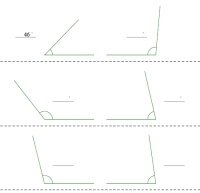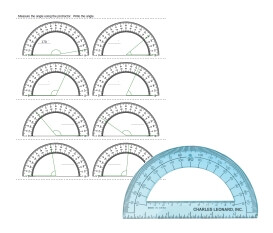Worksheets and No Prep Teaching Resources
Math Worksheets

# Angle Worksheets

For a new angle on teaching angles, try these great worksheets! Students will love these remarkable lessons about angles, including measuring with a protractor, rays, and classifying various kinds of angles, all the way up to the Pythagorean Theorem. Perpendicular and parallel lines are also explored and covered in creative mixed review sheets.Using a Protractor and AnglesAngle activities using a protractor

Lines and Line Segments

Rays and Angles

Name each figure using letters and symbols
Draw and label an example of each
Classify each pair of lines as parallel, intersecting, or perpendicular
Classify each angle as acute, obtuse, straight, or right
Classify polygons
Classify triangles
Classify triangles according to lengths
Circles
Plane Figures Mixed Review

Naming angle pairs (easy)
Naming angle pairs (pictures with 4 angles)
Angle pairs: Find the unknown angle measure
Naming adjacent, complementary, supplementary, and vertical angles
Find the unknown angle measure (pictures with 5 angles, one center)
Naming alternate interior/exterior angles, vertical angles, and corresponding angles
Find the unknown angle measure (traversals with parallel lines)
Lines and Angles Mixed Review

Basics of Geometry
Points, Lines, and Planes (part 1)
Points, Lines, and Planes (part 2)
Segments, Rays, and Distance
Angles (part 1)
Angles (part 2)
Parallel Lines
Angles of Triangles
Angles of Polygons
Basics of Geometry Mixed Review

Perpendicular and Parallel Lines
Lines and Angles
Proving Parallel or Perpendicular
Properties of Perpendicular and Parallel Lines
Perpendicular and Parallel Lines Mixed Review

Circles
The Basics of a Circle
Tangents to Circles
Arcs and Angles
Proofs Involving Arcs and Chords
Circles Mixed Review

Right Triangles
Similar Right Triangles
The Pythagorean Theorem
Special Right Triangles
Right Triangles Mixed Review

Trigonometry
Using a calculator (sin, cos, tan)
Using a calculator (inverse functions)
Find the tangent of one point
Tangent: Find the value of x
Find the sine of one point
Sine: Find the value of x
Find the cosine of one point
Cosine: Find the value of x
Mixed sine, cosine, and tangent
Mixed: Find the value of x
Fill in the missing angle
Angles of rotation: Coterminal angles
Angles of rotation: Reference angles
Angles of rotation: Coterminal and reference angles
The Law of Sines: Find one unknown
The Law of Sines: Find all unknown sides and angles
The Law of Cosines: Find one unknown
The Law of Cosines: Find all unknown sides and angles
Mixed review of the law of sines and cosines (one unknown)
Mixed review of the law of sines and cosines (find all unknowns)
Trigonometry Mixed Review

Coordinate Geometry
Graph the Line Segment
Find Area of a Triangle [given 3 points]
Find Area of Trapezoids
Review of Find Area
Find the Slope of a Line [given 2 points]
Determine if 2 lines are Parallel
Write Equation for a Line [given m and b]
Find the slope and y-intercept from an Equation
Find an Equation [given m and a point on the line]
Find an Equation [given two points on a line]
Mixed Review: Find an Equation
Graphing Linear Equations [using x- and y- intercepts method]
Graphing Linear Equations [using slope intercept method]
Graphing Linear Equations [using any method]
Graphing Linear Equations [using any method]
Solve 2 Equations Graphically [solutions are small whole numbers]
Solve 2 Equations Algebraically
Using Subtraction to Find Area
Mixed: Find Area of Triangles, Parallelograms, Trapezoids, and Squares.
Find the Midpoint (Given 2 Points)
Find the Missing Point (Given a Midpoint and either A or B of Line AB)
Distance: Find the Distance Between Two Points
Distance: Between A and the Midpoint (Given Two Points)
Determine if points ABCD are a Quadrilateral.
Find the Slope and Length of a Line (Given Points A and B)
Determine Whether Lines are Parallel or Perpendicular
Given a Slope and Points, calculate the missing coordinate
Determine if the Points are Collinear
Given Collinear Points, Find Missing Coordinate
Slope of Parallel and Perpendicular Lines
Prove that a Triangle is a Right Triangle
Prove that a Quadrilateral is a Parallelogram
Prove that a Quadrilateral is a Rectangle
Prove that a Quadrilateral is a Square
Mixed from last 4 worksheets

Have a suggestion or would like to leave feedback?